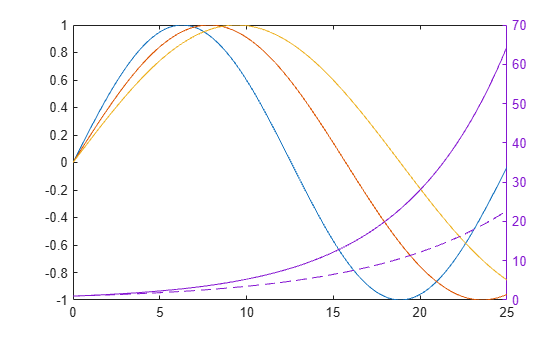Documentation

## Create Chart with Two y-Axes

This example shows how to create a chart with y-axes on the left and right sides using the `yyaxis` function. It also shows how to label each axis, combine multiple plots, and clear the plots associated with one or both of the sides.

### Plot Data Against Left y-Axis

Create axes with a y-axis on the left and right sides. The `yyaxis left` command creates the axes and activates the left side. Subsequent graphics functions, such as `plot`, target the active side. Plot data against the left y-axis.

```x = linspace(0,25); y = sin(x/2); yyaxis left plot(x,y);```### Plot Data Against Right y-Axis

Activate the right side using `yyaxis right`. Then plot a set of data against the right y-axis.

```r = x.^2/2; yyaxis right plot(x,r);```### Add Title and Axis Labels

Control which side of the axes is active using the `yyaxis left` and `yyaxis right` commands. Then, add a title and axis labels.

```yyaxis left title('Plots with Different y-Scales') xlabel('Values from 0 to 25') ylabel('Left Side') yyaxis right ylabel('Right Side')```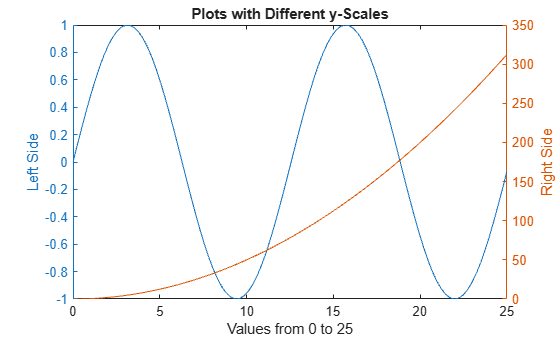### Plot Additional Data Against Each Side

Add two more lines to the left side using the `hold on` command. Add an errorbar to the right side. The new plots use the same color as the corresponding y-axis and cycle through the line style order. The `hold on` command affects both the left and right sides.

```hold on yyaxis left y2 = sin(x/3); plot(x,y2); y3 = sin(x/4); plot(x,y3); yyaxis right load count.dat; m = mean(count,2); e = std(count,1,2); errorbar(m,e) hold off```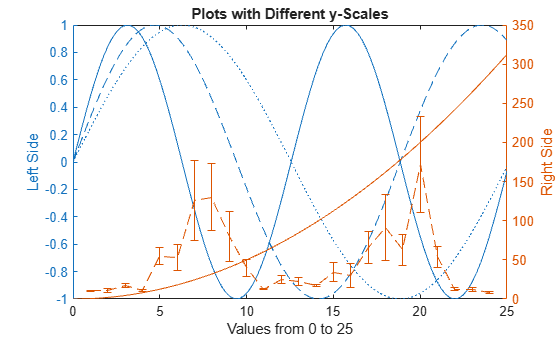### Clear One Side of Axes

Clear the data from the right side of the axes by first making it active, and then using the `cla` command.

```yyaxis right cla```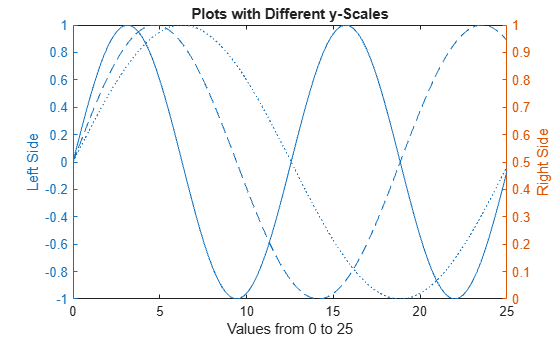### Clear Axes and Remove Right y-Axis

Clear the entire axes and remove the right y-axis using `cla reset`.

`cla reset`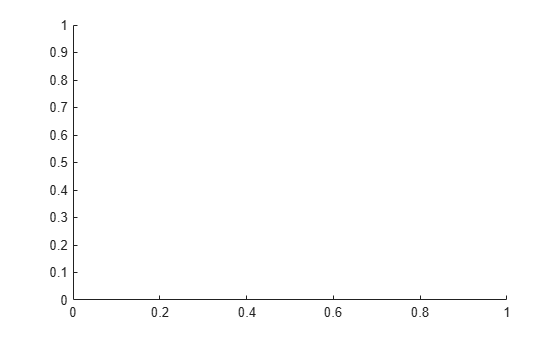Now when you create a plot, it only has one y-axis. For example, plot three lines against the single y-axis.

```xx = linspace(0,25); yy1 = sin(xx/4); yy2 = sin(xx/5); yy3 = sin(xx/6); plot(xx,yy1,xx,yy2,xx,yy3)```### Add Second y-Axis to Existing Chart

Add a second y-axis to an existing chart using `yyaxis`. The existing plots and the left y-axis do not change colors. The right y-axis uses the next color in the axes color order. New plots added to the axes use the same color as the corresponding y-axis.

```yyaxis right rr1 = exp(xx/6); rr2 = exp(xx/8); plot(xx,rr1,xx,rr2)```